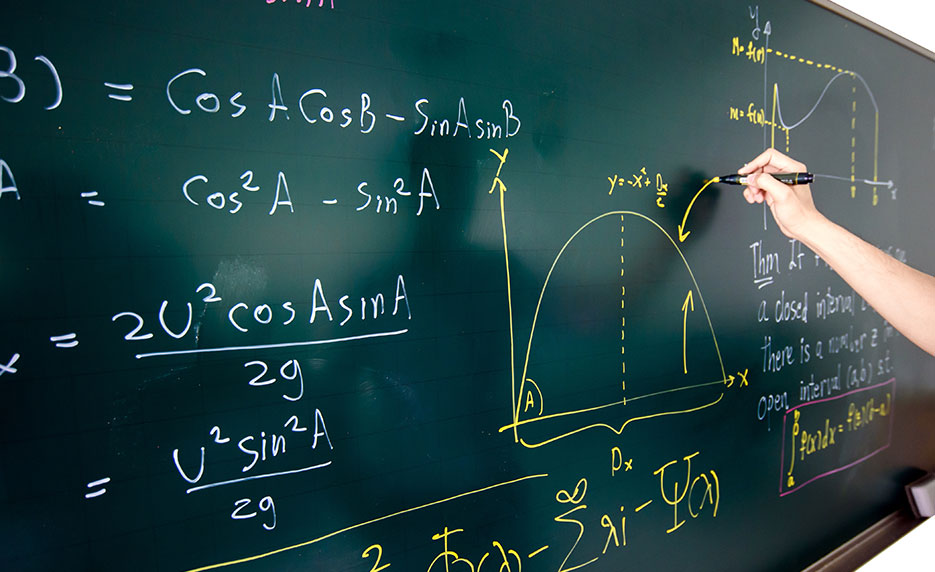# Introduction to Algebra (Self-Paced Tutorial)

Understand how algebra is relevant to every aspect of your daily life and become skilled at solving a variety of algebraic problems.

3 Months Access / 24 Course Hrs
• Details
• Syllabus
• Requirements

## Details

This online math course integrates mathematics, specifically algebra with many other areas of study, including history, biology, and geography. You will develop a rich understanding of math while you study algebra right here in a relaxed and supportive learning environment. Its emphasis on practical math applications of your new-found algebraic skills will help you learn math reasoning in a real-world context. As a result, you will acquire a wide variety of basic math skills that will help you find solutions to almost any math problem.

Math problem solving has never been made easier with this concise and straightforward course to help you understand some of the most important mathematical concepts of algebra: order of operations, units of measurement, scientific notation, algebraic equations, rational numbers, accounting concepts like calculating simple interest, and more.

By the time you finish this online math course, you will understand how algebra is relevant to every aspect of your daily life!

##### Enrollment Options:
Self-Paced
3 Months Access Course Code: T9316
No Instructor, Start Anytime
\$115.00 USD

## Syllabus

Algebra: Here to Make Life Simpler

Your first lesson introduces Al-jabru, the Arabic word for algebra that means the science of restoration and canceling. You will search for algebraic solutions and learn definitions, numeric properties, and basic operations that originated from the ancient lands where papyrus was used instead of paper.

How Do You Measure Up?

How do calories contribute to weight gain? You will answer this and other questions in this lesson. You will learn the different physical quantities, their units of measurement, and why they're important. You will also learn the difference between mass and weight, and density and weight density.

Evaluation Time Again

This lesson focuses on evaluation, but not the type of evaluation your boss might give you. You will learn about algebraic expressions by using the order of operations and the distributive property translation. You will also practice translating word problems into numerical ones.

Formula: Another Name for Equation

In this lesson, you will learn formulas for basic shapes: squares and rectangles, triangles, circles, spheres, cylinders, and cubes. You may be thinking, "Is this also a geometry course?" In a way you're correct—some aspects of geometry involve formulas, which people use in everyday life.

Balancing Acts: Equations

This lesson will teach you how to balance equations with formulas, such as those used to calculate interest, distance, and temperature. You will also learn how to translate these types of verbal equations into numerical ones. So, this is a chance to hone your mathematics reading skills, too.

Investments, Mixtures, and Percentage Changes

In this lesson, you will calculate problems involving percentages, such as how much to invest at different interest rates to obtain optimum interest, and you will learn how to determine a specific quantity of a component of a mixture or solution given specific concentrations.

Equate It in Words

The more you practice, the better you will be able to speak the language: the math language. Practice makes perfect! So, in this lesson, you will calculate populations, averages, lengths, and widths. You will also learn how to calculate consecutive integers, including consecutive odd and even integers.

Proportions and Variations

In this lesson, you will learn ratios and how to apply them to probability and odds. You will also find out how quantities vary in equations, whether directly or indirectly, and how to apply these variations to scientific laws and equations involving weight, area, and equations of lines.

Uniform Linear Motion

This lesson brings you outdoor to solve equations of uniform linear motion, with activities such as jogging, boating, and traveling. You will learn how to calculate distance, rate (speed), and time while going in the same direction, opposite directions, and with wind and water current.

Solving Rational Equations

This lesson focuses on using the formula that employs the same concept as the uniform linear motion equation. You will learn to calculate how long it takes to finish a job, how much of the job is done, and at what rate the job is done, depending on the number of people doing the job.

Algebraic Rational Equations

Discover some of the natural laws that govern our universe and delve into other physics concepts such as resistance, conductance, focal length, and forces while you learn some history of D-Day.

Potpourri of Algebraic Equations

Potpourri, anyone? Test various problem-solving skills while you meet General Sherman and experience the gloominess of the Great Depression.

## Requirements

Prerequisites:

There are no prerequisites to take this course.

Instructional Material Requirements:

The instructional materials required for this course are included in enrollment and will be available online.

Requirements:

Hardware Requirements:

• This course can be taken on either a PC, Mac, or Chromebook.

Software Requirements:

• PC: Windows 8 or later.
• Mac: macOS 10.6 or later.
• Browser: The latest version of Google Chrome or Mozilla Firefox are preferred. Microsoft Edge and Safari are also compatible.